## Section 6 -  Interaction

### 6.1 Direct Manipulation of Reals

So far we have already interacted with our data and our displays in various ways. In this section we introduce a new interaction technique, the direct manipulation of data. By using a DirectManipulationRenderer we will by able to change the data value simple by dragging its depiction on the display. In other words, suppose you have an object at a given location. We will represent it by a point, or a cursor. The object's data are, for example, its position, which is given by a pair of coordinates. We shall then change the position value by dragging the cursor around the display. In this section we shall restrict ourselves to 2D, but in a later section we will use 3D displays.

We start off by defining the quantitities which are going to by drawn on the axes. We will call them northing and easting, and have created them as usual:

```
easting = new RealType("easting", SI.meter, null);
northing = new RealType("northing", SI.meter, null);
```
(These are not to be confused with the system intrinsic RealType.Latitude and RealType.Longitude.)

Our object, henceforth refered to as cursor, has a pair of coordinates attached to itself. These coordinates are cursor's data, which will be able to change by means of direct manipulation. The data are represented by a pair (tuple) of values. We are dealing with a tuple of VisAD Reals. Each coordinate component is given by a Real. A Real is a VisAD Data class, and we need two such objects, nicely packed in an array:

```
Real[] reals  = { new Real( easting, 0.50),
new Real( northing,  0.50) };
```
Each coordinate component has a value of 0.5. As said, we pack those inside a RealTuple, which we had declared and created with
```
RealTuple cursorCoords;

cursorCoords  = new RealTuple(reals);

```
This is already our final data object in the example. A RealTuple represents a vector of Reals. (Note: a mathematical vector, not a Java Vector.) Real is the class of VisAD scalar data for real numbers represented as double precision floating point values, which you can access with Real.getValue(). Double.NaN is used to indicate missing values. Real objects are immutable, which means that after constructing a Real you can only reassign its value by reconstructing it. Note that there are other constructors for a RealTuple. Please consult the VisAD Javadoc for more information.

Of course, we will also need a display and some ScalarMaps as well as a DataReference object to link display with teh cursor. First we create the DataReference and set it with the cursor's data, that is, the cursorCoords:

```
cursorDataRef = new DataReferenceImpl("cursorDataRef");

cursorDataRef.setData( cursorCoords );
```

There's no mystery about the display and its maps:

```
// create display
display = new DisplayImplJ2D("display0");

// create maps
lonMap = new ScalarMap( northing, Display.XAxis );
latMap = new ScalarMap( easting, Display.YAxis );

```

We are almost done. The next step would be to add the data reference to the display. However, if we do that, the cursor will be drawn in white, which is OK, but will also be dran with one pixel size, which is not OK for most displays. So we take the oppotunity to create an array of ConstantMaps, to set some cursor's attributes:

```
ConstantMap[] cMaps = { new ConstantMap( 1.0f, Display.Red ),
new ConstantMap( 0.0f, Display.Green ),
new ConstantMap( 0.0f, Display.Blue ),
new ConstantMap( 3.50f, Display.PointSize )  };
```
That is, the cursor will be drawn in full red, without green or blue, and will have a size of 3.5 pixels. Now we are ready to add the data reference to the display:
```
display.addReferences( new DirectManipulationRendererJ2D(), cursorDataRef, cMaps );
```
Note the method's name, it is addReferences and not addReference as we've seen so far in this tutorial. Also note that the method takes as parameters a DirectManipulationRendererJ2D as well as the usual DataReference and array of ConstantMaps. Next we pack the display inside a JFrame and show it, as we've done so many times before.

Running the program above (the complete code is available here) with "java tutorial.s6.P6_01" generates a window like the screen shot below.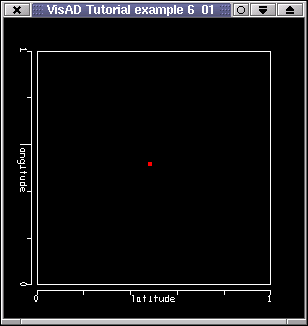Clicking the right mouse button on the cursor and dragging it will move the cursor about. Note that by doing this, you will by changing the value of cursorCoords. In this example this is not very exciting. You're are "just" moving a cursor about. If, however, you have other data linked to or dependent on cursorCoords, with dragging you can then change those other data, too. You should also try changing the ConstantMaps, or even setting it to null, in which case you should get a minute white cursor. It still draggable, though. That is, if you can click on it!

In practice you'd use the DirectManipulationRendererJ2D to change other data and/or provide more interactivity to your application. In the next section we shall do something more useful with a "directly manipulatable" cursor.

### 6.2 Direct Manipulation of Reals and Function.evaluate()

Now that we know how to have interactive data objects on the displays, we shall try to do something interesting and perhaps learn a few useful methods.

The starting point is the previous example. The major addition is the surface of section 3.1, which was defined as a FlatField. We have trimmed example P3_5 into a new class, called Surface. Surface has a method called getData, which returns the FlatField. The idea is to have the cursor floating over the surface, so that when we move the cursor, we get some information about the field. We are then ready to create the Surface object, and get its FlatField, which we called surfsField:

```
Surface surf = new Surface();
surfsField = surf.getData();

surfDataRef = new DataReferenceImpl("surfDataRef");
surfDataRef.setData(surfsField);
```
The last two lines above create and set a new data reference object for the surface. Later we will link the surface to the display with this data reference.

But how do we calculate the value of the function (FlatField) at the cursor position and when do we do that has yet to be explained. The "when" is simply every time the cursor changes. In other words, every time its data value changes. We use a CellImpl, whose doAction() method will contain code to evaluate the field at the given position. Linking the cell to the cursor's data reference will make sure doAction() gets called every time the cursor changes position. We create the CellImpl and implement its doAction() with:

```
CellImpl cell = new CellImpl() {
public void doAction() throws RemoteException, VisADException {

// get the data object from the reference. We know it's a RealTuple
RealTuple coords = (RealTuple) cursorDataRef.getData();

// then break the tuple down its components
Real lon = (Real) coords.getComponent(0);
Real lat = (Real) coords.getComponent(1);

// print the value of each component
System.out.println("Cursor at: (" + lon.getValue() + ", " +
lat.getValue()+ ")");

// Evaluate the value of the function
// temperature = f( easting, northing)
// at the cursor position
Real tem = (Real) surfsField.evaluate(coords);

System.out.println("Temperature = "+ tem.getValue() );
}
};

```
In other words, when the method is called, we get the data object attached to cursorDataRef, which we know is a RealTuple, so we cast the Data to it and define coords as the new cursor coordinates. We then want to retrieve each coordinates component value, so with
```
Real lon = (Real) coords.getComponent(0);
```
we get the first component, easting. We do the same for northing and print the cursor's new coordinates, whose component double values we got with getValue(). Next we evaluate the value of the field at the cursor position. For that we use the method Function.evaluate(RealTuple domain). Remember, Function is an interface which is extended by the Field interface, which is implemented by FieldImpl which is extended by FlatField.) To put it in another way:
```
Real tem = (Real) surfsField.evaluate(coords);
```
evaluates the function at coords and return a Data object, which we cast to Real. in mathematical notation the FlatField surfsField represents temperature = f( northing, easting). Function.evaluate(coords) thus is the same as temperature = f( new_long_value, new_lat_value). Note that with another function, the returned data might be of a different type. But here we know it's a Real. After that we print the temperature value. Of course, we can't forget to add the cursor's data reference:
```
```
This will make sure doAction() gets called every time the data under cursorDataRef changes (when the cursor moves).

Note that the cursor is now white. We simply changed the ConstantMaps a little. We have also added an

```
rgbMap = new ScalarMap( temperature, Display.RGB );
```
to the display, to color the surface according to the temperature value.

You can see these changes in the code available here. Run the example with "java tutorial.s6.P6_02" and you'll see a window like the picture below.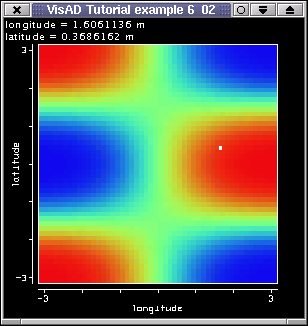The cursor dynamic matches that of the previous examples. Clicking on the white cursor with the right mous button and dragging it will run the code in doAction(). Information about the cursor's position and the temprature value at that point will be printed out (on the terminal window).

Before we move on there's a point or two to be considered. The VisAD FlatField represents a finite set of (temperature) samples. The function this Field represents is, however, continuous. In practice, this means that the values you're seeing printed out are "interpolated" values. You can actually choose the interpolation method. The method Function.evaluate() has other forms. For example, the method

```
evaluate(RealTuple domain, int sampling_mode, int error_mode)
```
Will evaluate the Function at domain with non-default modes for resampling and errors (which are Data.WEIGHTED_AVERAGE and Data.NO_ERRORS). Availbable interpolation (sampling) modes are Data.WEIGHTED_AVERAGE and Data.NEAREST_NEIGHBOR and other available error modes are Data.INDEPENDENT and Data.DEPENDENT. Just a final note before we move on: if you move the cursor outside the surface, that is, where the function is not defined, you will get missing data, in form of NaN's (Not a Number), being returned.

[Top] [Home] [Back]

### 6.3 Direct Manipulation of Reals and Function.resample()

In the previous section we evaluated a function at a given domain value. We shall now do exactly the same, but will take another approach. We mentioned Function.evaluate() interpolates the function at the given location. VisAD offers methods for interpolation "as we know it". That is, suppose you have two points, each of which has a value of some quantity atached to itself. If you want to calculate the value of this quantity somewhere between the points you talk about interpolation. Well, Function.evaluate() does that for you, providing you feed it with the right parameter (domain). Your domain, however, need not be a simple domain, like we had in the previous example. In this section we will use Function.resample(Set newDomainSet), or, more specififically, FlatField.resample() to change the domain set of our function.

We start off by thinking that our surface has a Linear2DSet with manifold dimension equal to 2. That is, it is a regular grid. If the 2D domain set had manifold equal to 1, then it'd represent a line in 2D space. Surely, if your set has just one point, than it does not represent a line, but just a single point, pretty much like our cursor so far. You can construct a 2D set with 1 point using:

```

Gridded2DDoubleSet(MathType type, float[][] samples, int lengthX)
```
Where lengthX = 1 and type is the domain type:
```

type = new RealTupleType(easting, northing);
```
samples is the location of the points, and length is the number of points (for us one point will do).

The idea is, whenever the cursor is moved, our Cell doAction() will execute and that means getting the cursor location, like before, and with this location, we will resample our 2D field (the surface) to a 2D point. We will finally print the value (temperature) we got, just like in the previous example.

```

RealTuple coords = (RealTuple) cursorDataRef.getData();
```
We then break the tuple down its components
```

Real lon = (Real) coords.getComponent(0);
Real lat = (Real) coords.getComponent(1);

```
and finally create the set:
```
int numberOfPoints = 1;
Gridded2DDoubleSet new2dSet = new Gridded2DDoubleSet( domain,
new double[][]{{x},{y}},
numberOfPoints);

```
Take some time to analyze how the array samples is organized:
```
samples = new float[ domainDimension ][ numberOfSamples];
```
If we had a line with multiple points in 2D, samples would be:
```
samples = {
{x1, x2, x3, ...},  // x values
{y1, y2, y3, ...},  // y values

};
```

Note that we chose a Gridded2DDoubleSet because the samples were already doubles. If we had floats we could have also chosen a Gridded2DSet. We are then ready to resample with

```

FlatField temporField = (FlatField) surfsField.resample(  new2dSet,
resampMode,
errorMode ); );
```
In other words, we resample the surface with a different 2D set, and now with non-default resampling and error modes. (Remember, the surface has a Linear2DSet but our new set represents a point in 2D space.) The method returns a FlatField whose domain set is the new Gridded2DDoubleSet. That is, it still represents a function temperature = f(easting, northing), also written as ((easting, northing) -> temperature ), but it has just one point as it domain set. We don't need this temporary field for long, so we get all temperature values stored in it:
```

double[][] tem1 = temporField.getValues();

```
Now we have all temperature values associated with this FlatField. That is, we have a single temperature value, stored in:
```

tem1
```
which we print. Still we calculate the temperature almost like we did in the previous example:
```
Real tem2 = (Real) surfsField.evaluate( coords,
resampMode,
errorMode );

```
but also with user defined resampling and error modes. The temperature values, calculated with both methods are printed out when the cursor is moved. Please run the code with "java tutorial.s6.P6_03" and you'll see a window like the picture below.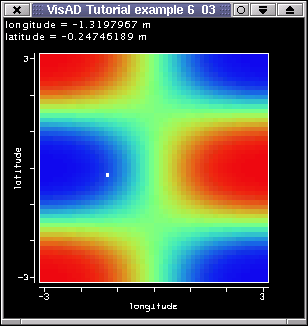There shouldn't really be any difference between the evaluated and the resampled value, although they are calculated in quite distinct ways. If you're feeling very brave, you can try changing resmpling and error modes to see if you get any differences between the values, just uncomment the appropriate lines in the code. Off course, evaluate() and resample() don't have to have identical modes. You could also experiment with the size of the surface. You could, for example, enter different dimensions directly in the Surface code, or even change the code to let the surface interpolate itself (downscale or upscale) before you display it. Note that, this is the "classical" way to interpolate, say, a line in VisAD. You simply create a larger domain set (by defining more points) and call resample() with this set as a parameter. Another example would be resampling an image so that its new size matches the size of another picture you want to compare.

So, the question left in the air is simply: when should I resample and when should I evaluate a function. Evaluate is simply to get the value of a field at a given location. That is, you are restricted to the current data structure. Resample, on the other hand, changes the data structure (but not the MathType!), which you can use in other different ways. For example, if you had a line crossing the field, then you could display the intersection of line with the surface as a graph of the temperature along the line. By feeding resample() with the "right" set, you can easily get this line. We'll do it next.

[Top] [Home] [Back]

### 6.4 An Interactive Line

In this section we'll show how to combine the interactive cursor with a line. The result will be a movable line, which will "sample" the surface. The actual (re-)sampling of the surface into a line will be done in the next section. Here we'll only create the line and make it movable.

We shall call our movable line the white line, and it is given by:

```
private Set whiteLine;
```

It will need its own data reference:

```
private DataReferenceImpl wLineDataRef;
```

The cursor in this example, however, doesn't need a pair of coordinates. It's position will simply be given by the northing value it is at. The reason for that is simply that we want the line to cut the surface in the horizontal direction. That is,the northing for a given line is constant. Our cursor position, so far given by a RealTuple is now given by a Real:

```
double initLatitude = 0.50;
cursorCoords  = new Real(northing, initLatitude);
```

To create the white line we use the method

```
private Set makeLineSet( double northingValue, int pointsPerLine )
{

// arbitrary easting end values of the line

double lowVal =  -4.0;
double hiVal =  4.0;

double[][] domainSamples = new double[pointsPerLine];

double lonVal = lowVal;
double increment = ( hiVal - lowVal )/ (double) pointsPerLine ;
for(int i=0;i < pointsPerLine;i++){

domainSamples[i] = lonVal;
domainSamples[i] = northingValue;
lonVal+=increment;
}

return new Gridded2DDoubleSet( domain, domainSamples, pointsPerLine);
}

```
The method takes the current northing and the number of points the line will have as parameters. We fix the easting min and max values to be -4 and 4, respectively. We then create the array which will hold the line samples (points) and loop over the number of points to set their values. Latitude is constant and easting gets incremented. The method returns a Gridded2DDoubleSet, our new white line.

This method will be called every time the cursor moves. But we also need to create the line for the first time:

```
int numberOfPoints = 100;
whiteLine = (Set) makeLineSet(initLatitude, numberOfPoints);
```
Let's not forget the data reference:
```
wLineDataRef = new DataReferenceImpl("wLineDataRef");
wLineDataRef.setData(whiteLine);
```

The new features in the doAction() are the lines

```
int nOfPoints = 100;
whiteLine = (Set) makeLineSet(latValue, nOfPoints);
```
which, just like above, will (re-)create the white line, and
```
wLineDataRef.setData(whiteLine);
```
which will reset the data, and, thus, will update the display. We also can't forget to
```
```

otherwise our diplay won't show the line.

Note that this time we have extended the array of ConstantMaps in order to be able to set a fixed cursor easting (x-axis) position:

```
ConstantMap[] cMaps = { new ConstantMap( 0.0f, Display.Red ),
new ConstantMap( 1.0f, Display.Green ),
new ConstantMap( 0.0f, Display.Blue ),
new ConstantMap( 1.0f, Display.XAxis ),
new ConstantMap( 3.50f, Display.PointSize )  };

```
The new ConstantMap( 1.0f, Display.XAxis ) means that the cursor will have a fixed x-value of 1.0, which puts the cursor on the box right hand side. (Remember, the VisAD display is originally a box of side equal to 2 and centered in (0,0,0).) If you uncomment this line, the cursor will be draw at (0, initialLatitude), that is, northing will change, but the easting value will always be the middle of the x-axis.

You can have a look at the code, run it with "java tutorial.s6.P6_04" and you'll see a window like the screen shot below.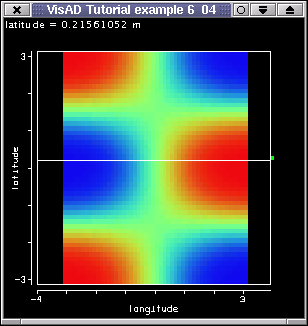Pressing the right mouse button on the cursor and dragging it will make the white line move. The cursor has now only one degree of freedom, northing. Longitude is fixed by the ConstantMap. When the cursor moves, doAction() is called, as usual. This method gets the current northing value, reconstructs the line at this new northing and resets the cursor's data reference to update the display. Well, everything quite simple. What if we create a new function, say a FlatField like the surface, just by resampling the surface with the white line Set? Let us try that next.

[Top] [Home] [Back]

### 6.5 Resampling a surface into a line

We now want to "cut" the surface with our white line and display the intersection between line and surface. In other words, in doAction() we will create a new FlatField by resampling the surface (also a FlatField) with the white line. The result, the temperature line, will be shown on another display.

We start of by declaring the temperature line and its data reference

```
private FlatField temperLine;
private DataReferenceImpl tLineDataRef;
```

Beacuse we will now have two display we changed our display variable into

```
private DisplayImpl[] displays;
```

Above there's no definition whether the displays are 2- or 3D. A DisplayImpl can be either. We will use two 2D displays, but in the next section we will include code for a 3D display.

After we create the white line exactly as we did above, we can create the temperature line for the first time:

```
temperLine = (FlatField) surfsField.resample( whiteLine);

```

Let's take some time to analyze the line above. temperLine is a FlatField create from the resampling of surfsField, also a field itself. The resampling parameter is the white line Set, whose domain is the same as the surface's:

```
(easting, northing)
```
temperLine is not a line like the white line. The white line is a "simple" line (that is, it's only a Set), whereas temperLine is a more complex line. In fact, temperLine represents the function
```
( (easting, northing) -> temperature )
```
exactly in the same way the surface does, only that the temperLine function is sampled along a line. We could also create a Linear2DSet (or a Gridded2DSet with manifold dimension = 2) and feed resample() with it. The result would be a larger or smaller surface depending on the number of points this new set had. (Of course, the domain of this new set has to equal the domain of the original surface.) The point we want to make here is, the temperature line represents a function and the white line represents a Set (a simple line).

Before we move on, we can't forget the new data reference for the temperature line:

```
tLineDataRef = new DataReferenceImpl("tLineDataRef");
tLineDataRef.setData(temperLine);
```

The method doAction() has yet newer features:

```
temperLine = (FlatField) surfsField.resample( whiteLine);

tLineDataRef.setData(temperLine);
```
After we create the new white line (see previous example), we resample the surface with this new white line (Set) and get a new function returned, the temperature line at the cursor northing and along the easting values. The second line above simply tells the temperature line's data reference that the data object has changed, this will update the temperature line on the second display.

We now want two displays, so we do:

```
displays = new DisplayImpl;

for( int i = 0; i < 2;i++){
displays[i] = new DisplayImplJ2D("display" + i);
}

```
and also turn their axes on with
```
for( int i = 0; i < 2;i++){
GraphicsModeControl dispGMC =
(GraphicsModeControl) displays[i].getGraphicsModeControl();

dispGMC.setScaleEnable(true);
}
```

The first display will keep its maps, but we need new ScalarMaps for the second display. You cannot add the same map to different displays. But you can clone them:

```
```

That is, display1 has the same easting (x-axis) and temperature (rgb-color) maps as display0. Note that you can get the maps of a display with:

```
Vector mapsVec =  displays.getMapVector();
```
```
for( int i = 0; i < mapsVec.size();i++){

ScalarMap sm = (ScalarMap) mapsVec.get(i);
}

```

Next we add the cursor's, the surface's and the white line's data references to display0 and the temperature line's data reference to display1. This last action is done with:

```
ConstantMap[] tLineMaps = { new ConstantMap( 2.0f, Display.PointSize )  };

```
That is, first create ConstantMaps for larger points and then add the temperature line's reference with those ConstantMaps.

The two displays are added to our JFrame with:

```
jframe.getContentPane().setLayout(new GridLayout(1,2) );
```

where the first line of code is the interesting: we need to set a nicer layout, so that the displays will grow with the size of the JFrame, whose width we have doubled to acomodate two displays.

You can get the code here. If you run it with "java tutorial.s6.P6_05" and you'll see a window like the picture below.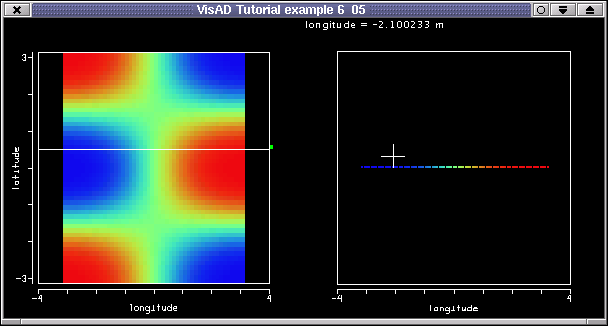The left side shows a display just like the one of the previous example. The right side has a new display, which shows the temperature line at the position of the white line on the left display. If you move the cursor up and down, the temperature line will be recalculted and redisplayed on te right. Note that the white line is somewhat longer than the surface's width. The temperature line is as long as the white line. So, what's happening on the edges, where the white line exists but where there's now surface? Remember what happened in section 6.2, when you moved the cursor out of the surface? The method Function.evaluate() returned NaN's. This is exactly what's happening with the edges of the temperature line. There, the temperature is not defined, and, thus, the temperature values are NaN's, which are not drawn.

Before we move on, we'd like to leave a question floating on the air. We've talked the whole time about temperature line, but we added this "line" to the display with an array of ConstantMaps. Isn't this contradictory? We'll look into this further.

[Top] [Home] [Back]

### 6.6 Resampling and Optimization issues

In the previous section we managed to cut the surface with an interactive line and display the intersection on another display. If you zoomed in the right hand side display, you will have noticed that the temperature line is not a line! It's a set of unconnected (easting, temperature) points. In this section we'll see why and also consider a few issues about optimization.

The starting point is the previous example. Everything is the same, except after we get the cursor data in the doAction(). Here, before we update the line, we want to check whether the cursor has moved significantly. We could build our own test by getting the cursor's old data and comparing with the new value, but we choose to use a method from the visad.util package:

```
// first get the current cursor northing
Real lat = (Real) cursorDataRef.getData();

// then test if value has changed more than 0.2
if( Util.isApproximatelyEqual( lat.getValue(), cursorCoords.getValue(), 0.2 ) ){

return; // leave method and thus don't update line
}
```

That is, we use the method Util.isApproximatelyEqual( double a, double b, double epsilon) to determine whether two numbers, the new value and the old value, are roughly the same. Here we chose epsilon (the absolute amount by which they can differ) to be an arbitrary 0.2. This means that the new lines are created after we move the cursor about 0.2 in the northing direction. If we move less than 0.2, the doAction() returns (that is, nothing happens). At the end of doAction() we can't forget to set the cursor data to be the current value:

```
cursorCoords = lat;
```

This is not the only optimization doAction() includes. Now we create the new white line with two points only, the start and the end point:

```
int nOfPoints = 2;
whiteLine = (Set) makeLineSet(latValue, nOfPoints);
```
Why we do this? Well, why not? We only need two points to define a straight line. Furthermore, the display won't have to recompute and then redraw all 100 points we had for the white line. For the temperature line we create a set with 100 points, like we had so far.
```
temperLine = (FlatField) surfsField.resample( makeLineSet(latValue, 100) );
```

We also add a new map to the second display:

```
displays.addMap( new ScalarMap( temperature, Display.YAxis) );
```

This will make the temperature line stretch out in the y-direction according to its temperature values. Now you must see that the temperature line is not a line. All the rest is done like in the prvious example.

You can get the code for this example here. If you run it with "java tutorial.s6.P6_06" and you'll see a window like the picture below.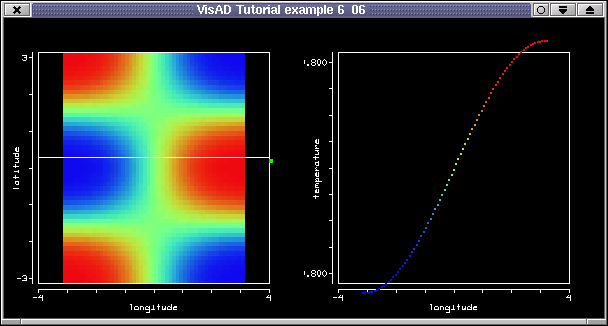Moving the cursor updates the white line and the data object on the second display. This update occurs only if we move the cursor by more than 0.2 of northing. The white line has now only two points, although one can't see any difference. What needs explanation is perhaps the fact that the so-called temperature line is made of points. The reason for that lies in the fact that the white line is a line in the 2D (easting, northing) space. The temperature line is also a line in this space. In the second display, though, we don't have this space, but a (easting, temperature) space, in which the values are points. If you need more convincing, then uncomment the following line in the code:

```
displays.addMap( new ScalarMap( northing, Display.ZAxis) );

```
In order to do this, you also need to change your DisplayImplJ2D to a DisplayImplJ3D:
```

displays = new DisplayImplJ3D("display" + 1);
```
This will make the second display a 3D display, and:
```
displays.addMap( new ScalarMap( northing, Display.ZAxis) );

```
which will add a northing map in the z-axis. Well, if you do so (the code lines above are already included in the code for this example) then you should see a 3D display on the right, showing a line with MathType
```
((easting, northing) -> temperature )

```
and maps like
```
easting -> XAxis
northing -> ZAxis
temperature -> YAxis

```

```
ConstantMap[] tLineMaps = { new ConstantMap( 2.0f, Display.PointSize )  };

```
has now no effect.

We answered a question but are then ready to throw two others in the air. First, how do we make a "real" line, that is a function like those seen in sections 1 and 2. (We mean a function like ( easting -> temperature ).) And secondly, is it possible to have a line in some direction other than only northing or only easting? Second answer first: yes, you could have any line; for example a line in the diagonal, as long as you feed your white line set with the correct values (which you'll have to calculate manually; an alternative would be to have two cursors, on on each line end, and from their coordinates, calculate the coordinates of a number of points between them). You could also have the whole thing in three dimensions. Just create the cursor with the adequate RealTuples and an adequate 3D line (or plane!).We'll do a couple of 3D examples shortly. First we turn our attention to generating a line function with the values we get from the resampling.

[Top] [Home] [Back]

### 6.7 Resampling a 2D Set into a 1D Set

In the previous examples we resampled part of a surface into a set of points. Those points do not form a connect line in the space defined by (easting, temperature). The points are, however, connected in the space defined by (easting, northing). You can confrim that if your display can accomodate all three variables, and we suggested switching the second display for a 3D display in order to make the topology evident. We're now interested in constructing a "proper" line. That is, we want to resample the surface, like we did before, but we want to use the data from the resampled Set to construct an object with MathType like ( easting -> temperature ) and a 1D Set for the domain. This is by no means difficult, but it requires some manual conversion of the data. In other words, we need to extract the temperature values to feed a new FlatField object, whose domain is the 1D Set.

The main idea in this example is the transformation of a 1D FunctionType into a 2D type. Of course we could create a 2D type by brute force, but VisAD offers us a convinient method for doing such a transformation. Consider the following String, which represents the MathType of a variable as a function of another:

```
String newFuncStr = "( easting -> temperature )";
```
So far our "lines" had a FunctionType like ( (easting, northing) -> temperature ). This is also the MathType of the surface. (Remember, the difference between the surface's domain and the object returned by the resampling the surface lies in the manifold dimension. The surface has manifold dimension equal to 2 and the resampled object - our collection of points - has manifold dimension equal to 1, that is, a line in the space defined by the RealTypes easting and northing.) To convert the MathType into the simpler type we can do:
```
FunctionType lineType = (FunctionType) surfsField.getType().stringToType(  newFuncStr ) ;
```
That is, lineType is our new type, created from the surface's type. It represents a variable (temperature) as a function of another variable (easting). (Whether the data object with this type will in fact be a line will depend on the domain set. But we'll make sure we'll choose an appropriate Set.) The static method MathType.stringToType( String newMathType ) could also be used, or we could have created the function with a FunctionType constructor. Finally note that we cast the returned MathType into a FunctionType.

We now turn our attention to the domain set of our newly created function. As said, we want a 1D Set, and we simply opt for a Gridded1DSet:

```
tLineSet = new Gridded1DSet( easting, new float[][]{lonSamples}, numberOfPoints);
```
The temperature line set is a 1D set, whose domain type is easting, whose samples is
```
float[][] lonSamples = whiteLine.getSamples(false);
```
simply extracted from the white line; the number of points in the set has also been given in the constructor.

We're ready to create the actual temperature line, using our freshly created MathType and Set, which are lineType and tLineSet , respectively:

```
temperLine =  new FlatField( lineType, tLineSet );
```
We now only need the actual temperature values and our FlatField will be ready to be displayed.
```
temperLine.setSamples( surfsField.resample( whiteLine).getFloats(false), false);
```
This is quite a bit in one line! We are setting the temperature values with values extracted from the surface. Those values are the floats from the surface (also a FlatField), resampled with the whiteLine. Note that we don't make a copy of those floats when we get them (the first false) nor do we make a copy of them when feeding the field (the second false). This field is ready to be displayed for the first time. We just can't forget to create a data reference for it and set the reference with the the field, like we've done many times before. The basic framework is ready. We need to implement this logic in the Cell.doAction, to create a new line everytime the cursor moves. The method is implemented as follows:
```
CellImpl cell = new CellImpl() {
public void doAction() throws RemoteException, VisADException {

// get the data object from the reference. We know it's a RealTuple
Real lat = (Real) cursorDataRef.getData();

// test if cursor postion (northing) has changed significantly
if( Util.isApproximatelyEqual(  lat.getValue(),
cursorCoords.getValue(),
0.1 ) ){

return; // leave method and thus don't update line
}
//...
};
```
That is, we get the data object associated with the data reference cursorDataRef and we check whether it's worth resampling the line, that is whether the cursor moved enough. If yes, we carry on and get the northing value, which we need to make the new white line. This new line has start and end points only, and we re-set its data reference, so that the display will be updated.
```
double latValue = lat.getValue();

// make a new line for display 1: will have only 2 points
int nOfPoints = 2;
whiteLine = (Set) makeLineSet(latValue, nOfPoints);

// Re-set Data, will update display
wLineDataRef.setData(whiteLine);

```
The next step is to create another "white line", but which won't be displayed. This line will be used to resample the surface. (Remember, the white line above is for display purpose only. The next "white line" is used as resample parameter.)
```
// now create a larger white line set to compute the temperature line

nOfPoints = 100;
whiteLine = (Set) makeLineSet(latValue, nOfPoints);

// get samples from white line
float[][] lonSamps = whiteLine.getSamples(false);

// create line function set
tLineSet = new Gridded1DSet( easting, new float[][]{lonSamps}, nOfPoints);

// fuction will have this type
String funcStr = "( easting -> temperature )";

// create Function (FlatField) and set the data
temperLine =  new FlatField( (FunctionType) MathType.stringToType( funcStr ), tLineSet );

temperLine.setSamples( surfsField.resample( whiteLine).getFloats(false), false);

```
This is almost exactly the same as we did just before the Cell.doAction. We don't draw the object we got from resample. We create a new function, based on a new MathType, create a new 1D Set and use the temperature values of the resampled object to feed the new function. The only diference to the approach before doAction is the use of the static method
```
MathType.stringToType( funcStr )
```
To create the new FunctionType from a String. As said, this is only an approach to illustrate the use of some VisAD data object's methods. In practice you would use stringToType to parse some user input in form of a String, perhaps when reading data from an ASCII file. VisAD's visad.data.text.TextAdapter does just that. Please refer to its code, which you'll find in your VisAD directory (in the subdirectory data/text). The final steps in the doAction() are to update the reference, which will update the temperature line on the right display and then to set cursorCoords to the current value, so that next time the cursor moves, we can check if the new value differs from the older by a significant amount.
```
// and update ist data reference -> will update display
tLineDataRef.setData(temperLine);

// assign current cursor position to old cursor position
cursorCoords = lat;

}
};

```
In fact, this example has brought so far, nothing new. We have just combined data data obejcts of diferent dimensions and have used the temperature values of one, to feed the other data object. The basic logic was really taken from the previous examples. There's still time to add something useful and enhance our mini application::
```

```
Well, we can't really claim we're introducing something new. We saw the VisADSlider back in chapter 5 (see for example section 5.7). The line above creates an instance of the VisADSlider. The parameters are a data reference, minimum, maximum and start values for the slider, the RealType easting and the label the slider will have. The really interesting thing about this slider is that, when the data object linked to the data reference cursorDataRef changes, the slider will change accordingly. Tat is, the slider accompanies the cursor and vice-versa.

Another little modification is the use of ConstantMaps to define some attributes of the white line:

```
ConstantMap[] wLineMaps = {  new ConstantMap( 1.0f, Display.Red ),
new ConstantMap( 1.0f, Display.Green ),
new ConstantMap( 1.0f, Display.Blue ),
new ConstantMap( 3.50f, Display.LineWidth )  };

```
The color maps are somewhat redundant, as they define a white color for a line which already was white. But you are free to define your own favorite color by changing the values of the components. (Bear in mind that those values must be floats between 0 and 1.) The last maps sets the line width. You'll notice that the line is now slightly wider. The array of ConstantMaps serves as parameter when adding a data reference to a display, as we know:
```

```
The very last changes regard GUI layout. We need a diferent LayoutManager to accomodate the two displays as well as the slider in our JFrame. This is done with the following lines.
```
JFrame jframe = new JFrame("VisAD Tutorial example 6_07");
jframe.getContentPane().setLayout(new BorderLayout());
JPanel dispPanel = new JPanel( new GridLayout(1,2) );

// Set window size and make it visible

jframe.setSize(600, 300);
jframe.setVisible(true);

```

You can see the complete code here. If you run it with "java tutorial.s6.P6_07" and you'll see a window like the picture below.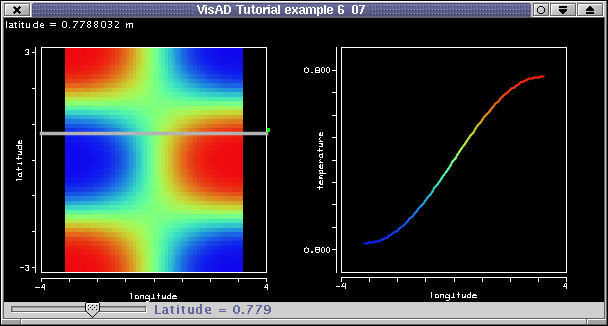This example follows the pattern of the last few examples. The real difference is in thetemperature line on the right display. It is in fact a line, just like we had promised. The other little but nice difference is the addition of the slider. The slider provides an alternative way of moving the cursor and, therefore, the white line With the slider youcan also see at which northing value the white line is in.

[Top] [Home] [Back]

### 6.8 Yet More Interaction with a VisADSlider

In the previous section we changed the MathType of the temperature line so that is was draw as a line. We also added a VisADSlider as an alternative way of interacting with data objects. In this section we shall not be so bold. We'll take it easy and won't change the previous example very much.

The encourage to use other sets Util.isApproximatelyEqual( nPoints.getValue(), ((Real) nPointsRef.getData()).getValue(), 2 ) add slider ConstantMap[] tLineMaps = { new ConstantMap( 2.0f, Display.LineWidth ) };

```

```

You can get the code here. If you run it with "java tutorial.s6.P6_08" and you'll see a window like the picture below.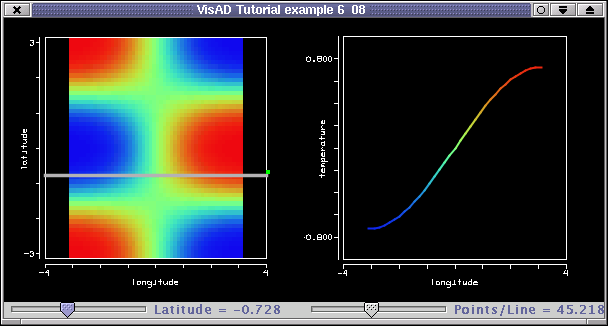### 6.9 Interaction in 3D

This example is a 3D version of the previous example. But whereas in the latter a cutting line is used to resample a plane into another line, the current example shows how a slicing plane is used to resample a cube into another plane. That is, the intersection between a (grey) plane and the cube is displayed on another 3D display.

Being a 3D application means that main difference to the previous example is the domain

```
domain3D = new RealTupleType(easting, northing, altitude);
```
created from three RealTypes. The 3D data (the one that will be resampled) is now a cube, available as a separate data class, Cube.java. The cube is a wrapper for a FlatField, whose domain set is a Linear3DSet, and whose range is temperature. (The domain type of the cube has been chosen so as to match that of the current example, the domain3D.) The VisAD data object is extracted from a Cube object by calling aCube.getData(). In the current example, the cube is created and linked to its data reference with the following calls:
```
Cube cube = new Cube();
cubeFF = (FlatField) cube.getData();

cubeDataRef = new DataReferenceImpl("cubeDataRef");
cubeDataRef.setData(cubeFF);
```
As said, the cube will be sliced by a grey plane, created by the following function from a initial altitude value and with a certain number of points:
```
greyPlane = (Set) makePlaneSet(initAltitude, numberOfPoints*numberOfPoints);
```
The function makePlaneSet() creates a flat three-dimensional surface at a given altitude value. The function uses the constructor:
```
Gridded3DSet( domain3D, domain2DSamples, ptsPerDim,ptsPerDim);
```
which is a 3D set with manifold dimension = 2, that is a surface in 3D. (Were it manifold dimension = 1 or manifold dimension = 3, it would be a line or a parallelpiped, respectively. Please consult the Javadocs, for further information.)
The samples, called domain2DSamples are in a float[pointsPerPlane] array, and are for the z dimension (altitude) all equal to initAltitude. In the x and y (easting and northing) directions, the samples are extracted from a temporary 2D set:
```
Linear2DSet tempor2DSet = new Linear2DSet(domain2D, lowVal,hiVal,ptsPerDim,lowVal,hiVal,ptsPerDim);
domain2DSamples = tempor2DSet.getSamples(false);
domain2DSamples = tempor2DSet.getSamples(false);
```
Please refer to the code for further details.
The rest of this example is almost like example P_08. The only difference being the 3D dispplay (on the right), and that the slicing object, the grey plane, is made grey and 75% opaque by the following constant maps:
```
ConstantMap[] wLineMaps = {  new ConstantMap( 0.75f, Display.Red ),
new ConstantMap( 0.75f, Display.Green ),
new ConstantMap( 0.75f, Display.Blue ),
new ConstantMap( 0.75f, Display.Alpha ) };
```

You can get the code here. If you run it with "java tutorial.s6.P6_09" and you'll see a window like the picture below. Moving the altitude slider will update the grey plane and the white cursor. Conversely, if you drag the white cursor with the right mouse button, the grey plane ad the alitude slider slider will by updated.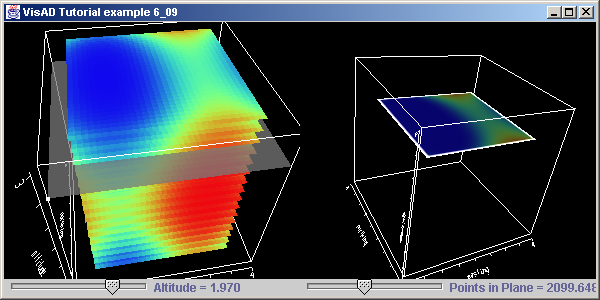On the right, the resulting plane will be shown, at the right altitude, on a 3D display. You can use the slider on the right to change the number of points on the temperature plane. For a small number of points, the program will run faster.

In the next example we shall add a few GUI elements, and add some more functionality to the this example, but without changing any of the VisAD data objects or its functions.

### 6.10 More Interaction with a GUI

This example builds up on the last one, introducing nothing really new, but adding a few GUI elements, and a couple of new features. So we describe this new example using a top-down approach. That is, run the example with "java tutorial.s6.P6_10" and refer to the window (or the image below) when reading the text below.

First thing you'll notice are the SelectRangeWidgets on the lower left hand side. For them to work, we need to create and add the following maps:

```
rangeX = new ScalarMap(easting, Display.SelectRange );
rangeY = new ScalarMap(northing, Display.SelectRange );
rangeZ = new ScalarMap(altitude, Display.SelectRange );

```
The widgets are created with the following function:
```
private Component createRangeSliders(){
JPanel p = new JPanel();
p.setLayout(new BoxLayout(p,BoxLayout.Y_AXIS));
try {
p.add( new SelectRangeWidget( rangeX ) );
p.add( new SelectRangeWidget( rangeY ) );
p.add( new SelectRangeWidget( rangeZ ) );
}
catch (Exception ex) {
ex.printStackTrace();
}
return p;
}
```
That is, all three widgets live inside a panel, which is added to the frame, like any ordinary Java GUI component. Note that the range sliders allow you to slice the cube out, replicating the functionality given by this slicer example, but with a fraction of the effort needed to implement the slice.
The second thing you should notice is the "Link Displays" checkbox. When the checkbox is clicked, rotaing or moving the display on the left (called here the cube display), will replicate the action for the display on the right. This is done with calls to the displays' projection controls and by making the current program implement the DisplayListener interface:
```
public class P6_10 implements DisplayListener
```
We then need to implement the displayChanged(DisplayEvent e) method of this interface. Before explaining how this is done in the example, we ought to remind you to register P_10 as the handler of DisplayEvents fired up by the cube display. In other words, you must add P_10 (the "this" object) as a listener of events generated by the cube display:
```
```
(Remeber that displays is the cube display.) If you forget this - and I often do - nothing of the code inside displayChanged() will happen. By the way, this is how displayChanged() is implemented:
```
public void displayChanged(DisplayEvent e)
if (e.getId() == DisplayEvent.FRAME_DONE) {
displays.getProjectionControl().setMatrix(displays.getProjectionControl().getMatrix());
}
}
}
```
There are three important things to take notice in the method above: first, rotating and moving (including zooming in and out) is a DisplayEvent.FRAME_DONE event, so we only do something if the fired event is such; second, we have introduced a boolean variable
```
```
that gets turned on and off whenever the user clicks on the checkbox (See the createSyncCheck() method.); third, and this is where the action happens, we set the left display's projection matrix to be identical to that of the cube display. This is all done in the last statement.
Another feature in this example is the ability to switch the right display between the 3D and the 2D modes. This is done in the switchDisplay(boolean twoD) method. The method is somewhat long and shall be omitted here. Please refer to the code (it's commented!). However, the important points are highlighted.
1. Before rebuilding the display, get the scalar maps with
`Vector scalarMaps = displays.getMapVector();`
2. Clear the old display with
```displays.clearMaps();
displays.removeAllReferences();```
3. Remove the old display from the parent panel with
`dispPanel.remove(displays.getComponent());`
4. If the display should be 2D, use a 3D display with a TwoDDisplayRendererJ3D().
`displays = new DisplayImplJ3D("display1",new TwoDDisplayRendererJ3D());`
5. Iterate over the scalar maps' vector to re-add the old maps to the new display.
(For a "pseudo" 2D display, you'll have to check if the scalar map is not to Display.ZAxis)
6. Finally, re-add the display to the parent panel.
7. Some minor intermediate steps have been omitted. Also, the code to create the button is also omitted here. (See createButton() in P6_10.java.
Clicking on the "Switch to xD" button will change the display mode. Note that if the display's renderer is a visad.java3d.TwoDDisplayRendererJ3D() then rotating the cube display will create an interesting projection of the 2D display. Try it out! (If you plan to use a "real" 2D display, that is a Java2D-based one, you'll have to add checks in displayChanged(); the matrices of 2D and a 3D displays are not compatible, that is, they do not have the same size.
The last feature in this example is a button to reset orientation of the cube display. For brevity's sake we omit the code to create such a button. Important point to note is that in the corresponding actionPerformed() method the cube display (displays) is reset with the call
```
displays.getProjectionControl().resetProjection();
```

You can get the code here. If you run it with "java tutorial.s6.P6_10" and you'll see a window like the picture below.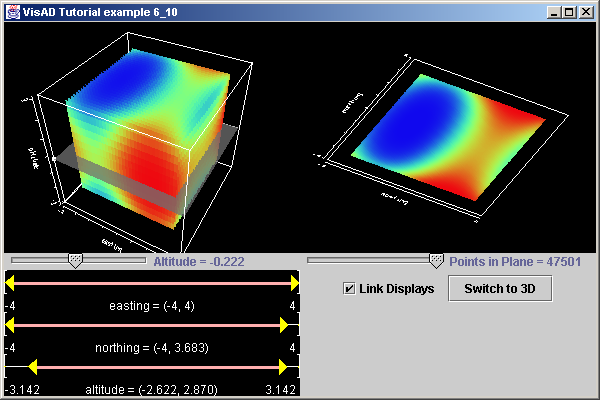Well, this is fine for now, as far as interaction is concerned. Off course, we have just started to scratch the surface of this topic in VisAD. But you might agree that the examples are getting too long, and there a need to avoid that. Here we could profit from a better design...or a change of topic! We leave you to change the design, add more features to your examples, and have fun.
In the next section we consider an altogether different topic: Text, Shapes and Vectors.

[Top] [Home] [Back]